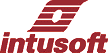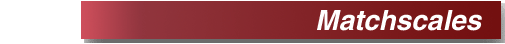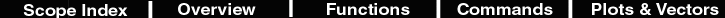Matchscales
 Description Makes the y-axis of all waveforms the same as the current (selected) waveform. Notice how the hotkey combination works (x Y y) to expand and link scales in both x and y axis. Hot Key y Script *&y Match all y-axis scales to that of the current trace. constants.max = maxscale(current) constants.min = minscale(current) alias refvec current nv = nextvector(null) while nv <> null if refvec <> nv setvec nv setylimits min max end nv = nextvector(nv) end setvec refvec